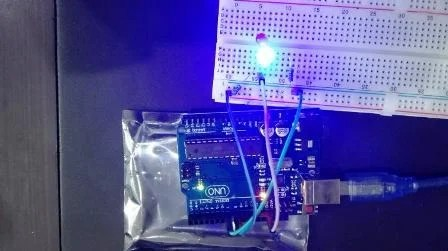# Led blinking with Arduino UNO R3

This is second tutorial on our series of tutorials on Arduino. In first tutorial on getting started with Arduino Uno R3 .I have provided you with overall overview of Arduino and how to program Arduino. In this article, you will learn how to use general purpose input output pins of Arduino and how to declare these digital pins as a output. Although these GPIO pins can also be used as a digital input pins. I will discuss it in next tutorial on how to use push button with Arduino. For this article, I will explain you digital pins through an example. Led blinking with Arduino UNO R3, this article give you brief review of how to use digital pins of Arduino UNO R3 as an input or output. I have also discussed how to use Proteus to learn Arduino UNO R3 if you don’t have its hardware board and how to write your first program or sketch for Arduino UNO R3. In this project two LEDS are connected with digital input/output pins of Arduino UNO R3. Arduino will turn on only one LED at a time and other will remain off for that time.Note : if you don’t know about pins of Arduino UNO R3 , first read following article.

### Circuit diagram of LED blinking in proteus

Circuit diagram led blinking with Arduino is shown below. Arduino have thirteen GPIO pins and 5 analog pins. But these five analog pins can also be used a digital pins.  To use these analog pins as digital pins, you just need to define them as digital through PinMode function which I will explain in later part of this article.  Arduino development board also has on board light emitting diode connected with pin number 13.  So you can also use this for this LED blinking example.

Two LEDS are connected with pin number 7 and 8 of Arduino board. Resistor R1 and R2 is used as a current limiting resistor.Maximum output voltage at Arduino pins is about 5 volt. By using maximum output voltage value one can easily calculate current limiting resistor value. Maximum current from these pins can never be greater than 30mA. Current output greater than 30mA damage Arduino board permanently. So by use maximum voltage and current limit, one can easily calculate resistance value.  For example

Vmax = 5 volt

Imax = 30mA

voltage drop across LED = 0.7 volt

Hence by using ohm law V = I * R

R =  5 – 0.7 volt / 30 m A = 150 ohm

But I recommend you to use resistor value more than calculated, to ensure safety of your Arduino board.

### Code of LED blinking using Arduino

Programming of Arduino is just like learning vocabulary of any language. You just have to learn name of some specific functions to use them in your program. If you know little bit about c programming like if/else statements, loops and switch statements, then you can easily learn Arduino programming by learning name of few specific functions. In this tutorial of led blinking, two functions are used. One is used to declare pin 7 and 8 as a output . You can check beginners introduction to Arduino programming article here.

Second function is used for making pin high and low. Functions names are given below :

pinMode(pin number, OUTPUT) : pinMode is name of function. Pin number and OUTPUT are arguments of function. For example, we have connected pin 7 and 8 with two leds and want to use pin 7, 8 as a output to blink LEDS. So above function can be used to declare pin 7 and 8 as a output pins as given below :

pinMode (7, OUTPUT)  i.e 7 is specified the pin number and OUTPUT tells Arduino that declare pin seven as OUTPUT pin.

pinMode (8, OUTPUT)  i.e 8 is specified the pin number and OUTPUT tells Arduino that declare pin seven as OUTPUT pin.

digitalWrite(pin number, HIGH/LOW) : digitalWrite is a name of function. This function make specified pin number in arguments either high and low. Remaining all the things in code given below are self explanatory.

```void setup()

{

pinMode(7, OUTPUT);

pinMode(8, OUTPUT);

}

void loop()

{
digitalWrite(7, HIGH);
digitalWrite(8, LOW);
delay(1000);
digitalWrite(7, LOW);
digitalWrite(8, HIGH);
delay(1000);
}```

But now the question is how to add above code in Proteus simulation ? To know about it watch the video given on above.  In above code delay() function is used to insert delay of 1000 ms between Light emitting diode blinking. if you still have any issue feel free to comment on this post. Check next post on how to use push button with Arduino ?

### 5 thoughts on “Led blinking with Arduino UNO R3”

1. Thanks for the tutorial. It explained the procedure and codes well. But my question is how to get Arduinoin Proteus I use version 7

• you can add library of arduino in proteus just download library of arduino in proteus and add in proteus models and library folder